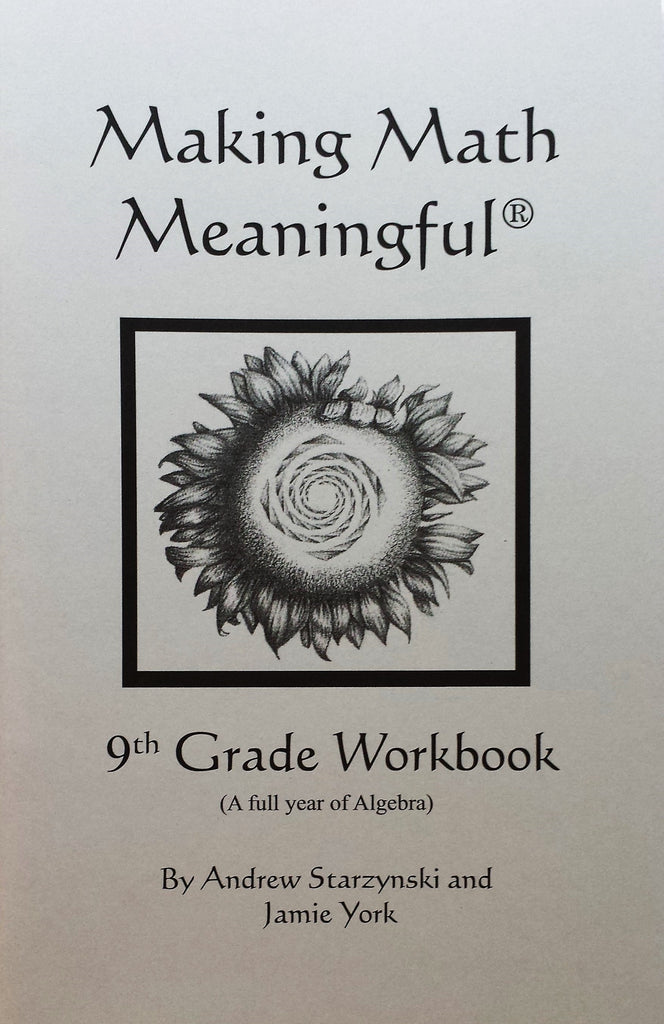\$ 8.95

In middle school, the students are introduced to some basic algebra concepts.  Now, in ninth grade, they are ready for a full year of algebra studies.  This important year of math forms the foundation for all future mathematics study — after all, algebra is the "language of mathematics."  There is a good deal of challenging work and learning to be done!

Units covered in this workbook are:

• Pre-Algebra
• Algebra Basics
• Exponents and Polynomials
• Factoring
• Word Problems
• Midyear Review
• Fractions and Square Roots